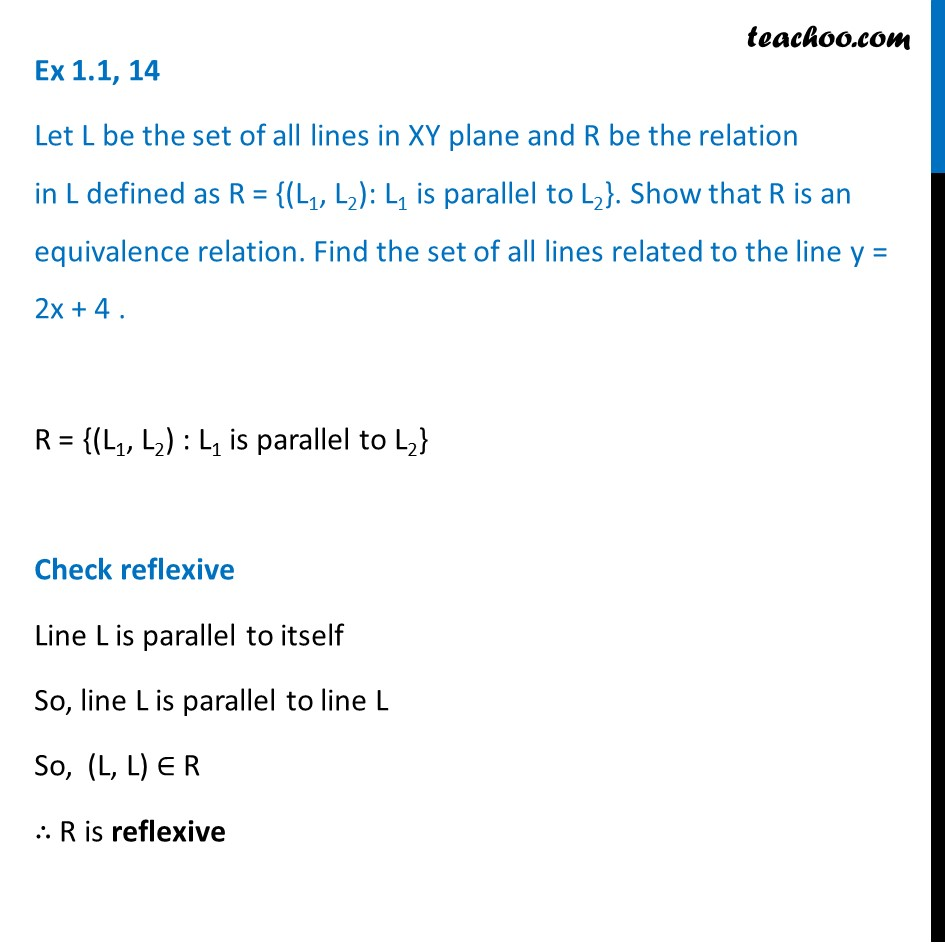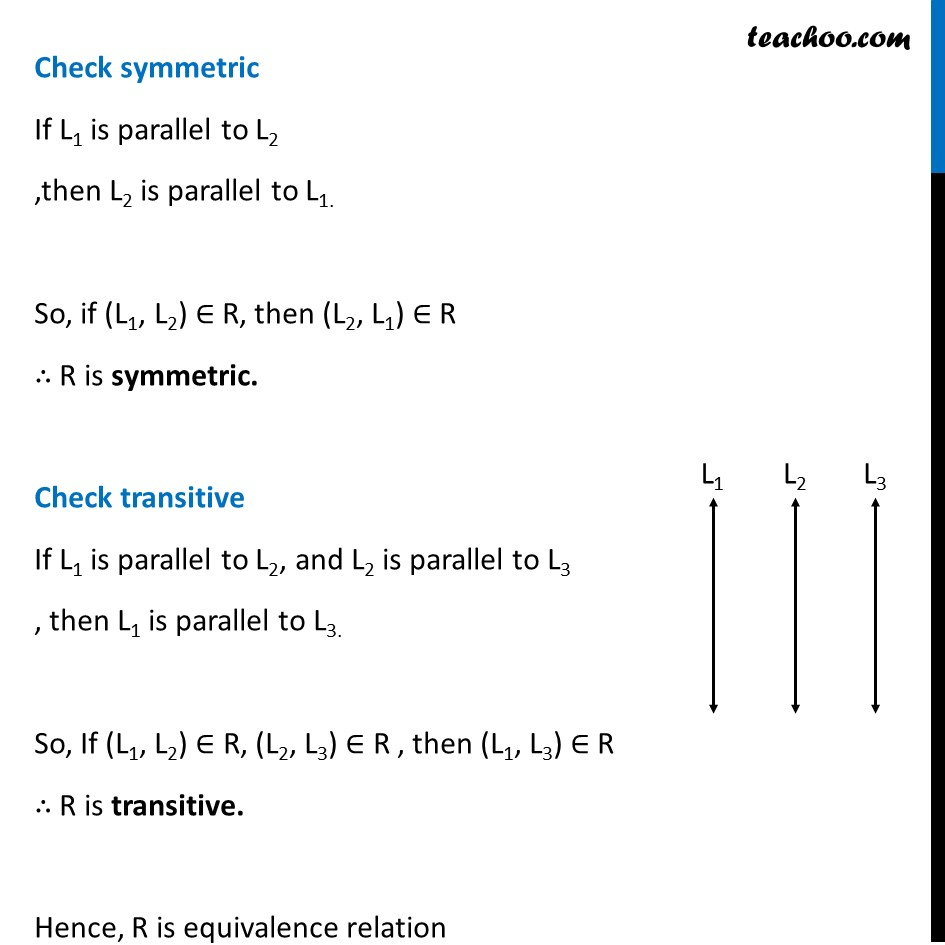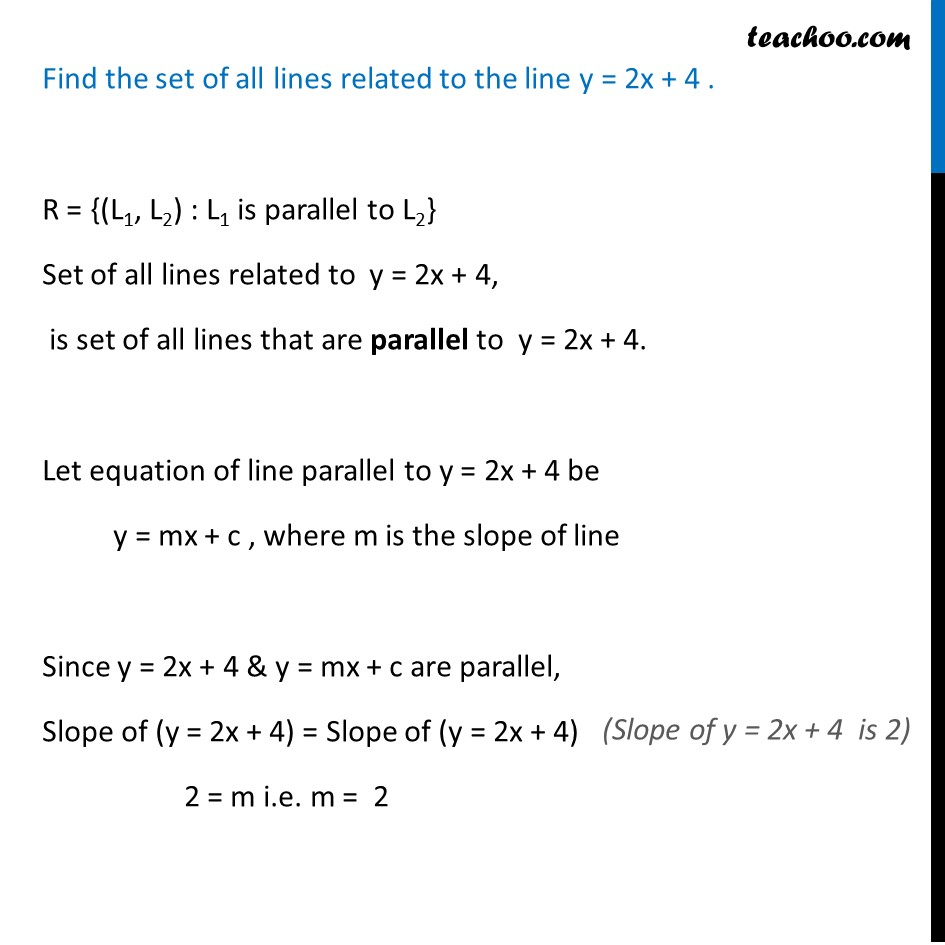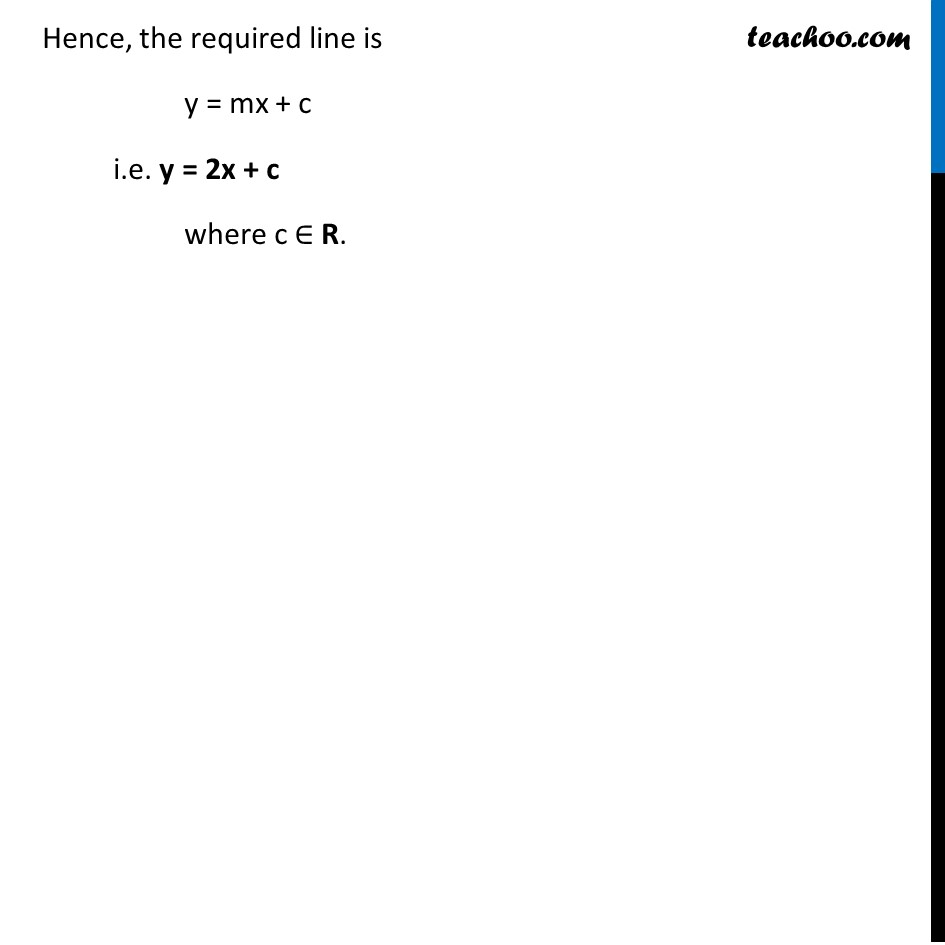Ex 1.1

Chapter 1 Class 12 Relation and Functions
Serial order wiseLearn in your speed, with individual attention - Teachoo Maths 1-on-1 Class

### Transcript

Ex 1.1, 14 Let L be the set of all lines in XY plane and R be the relation in L defined as R = {(L1, L2): L1 is parallel to L2}. Show that R is an equivalence relation. Find the set of all lines related to the line y = 2x + 4 . R = {(L1, L2) : L1 is parallel to L2} Check reflexive Line L is parallel to itself So, line L is parallel to line L So, (L, L) ∈ R ∴ R is reflexive Check symmetric If L1 is parallel to L2 ,then L2 is parallel to L1. So, if (L1, L2) ∈ R, then (L2, L1) ∈ R ∴ R is symmetric. Check transitive If L1 is parallel to L2, and L2 is parallel to L3 , then L1 is parallel to L3. So, If (L1, L2) ∈ R, (L2, L3) ∈ R , then (L1, L3) ∈ R ∴ R is transitive. Hence, R is equivalence relation Find the set of all lines related to the line y = 2x + 4 . R = {(L1, L2) : L1 is parallel to L2} Set of all lines related to y = 2x + 4, is set of all lines that are parallel to y = 2x + 4. Let equation of line parallel to y = 2x + 4 be y = mx + c , where m is the slope of line Since y = 2x + 4 & y = mx + c are parallel, Slope of (y = 2x + 4) = Slope of (y = 2x + 4) 2 = m i.e. m = 2 Hence, the required line is y = mx + c i.e. y = 2x + c where c ∈ R.